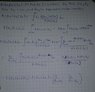# Expectation of E[X|X>Y]

• I
mertcan
Hi, Initially X and Y are exponential random variables with rate respectively $$\mu \lambda$$, and I am aware that E[X|X>Y] is obtained using joint distribution but I can not build up the integral structure, I intuitively think the result is just 1/mu, but I can not prove it to myself could you help me about that and building the integral structure?

Homework Helper
Gold Member
Start by writing the double integral that calculates the unconditional expectation ##E[X]##. Use ##x## as integration variable for the outer integral, and ##y## for the inner integral. Once you've done that, only a minor adjustment is needed to the inner integration limits to turn it into the conditional expectation ##E]X|X>Y]##.

mertcan
hi, I tried to do my work related to E(X1|X1<X2)*P(X1<X2), and X1, X2 are exponential random variables with rate respectively $$\lambda, \mu$$ I found a answer but I think it is wrong so could you tell me which part of my work is wrong?? ( I also looking forward to your answers @andrewkirk @Ray Vickson :) )

#### Attachments

•my work.jpg
37.5 KB · Views: 394
Homework Helper
Gold Member
Did you read my post? I told you that you need a double integral. Why have you tried to do something using only a single integral? The image you posted is too dark and smudgy to make out in detail what it says but even at a glance one can see that it only has single integrals, not double integrals.

mertcan
@andrewkirk @Ray Vickson I upload my work 2 ,let me express again that E(X1|X1<X2)*P(X1<X2), and X1, X2 are exponential random variables with rate respectively $$\lambda_1,\lambda_2$$I found a answer but I think it is wrong so could you tell me which part of my work is wrong?? ( by the way I did my best to make it not dark, when I upload, the top and bottom parts get dark a little )

#### Attachments

EngWiPy
Hi, Initially X and Y are exponential random variables with rate respectively $$\mu \lambda$$, and I am aware that E[X|X>Y] is obtained using joint distribution but I can not build up the integral structure, I intuitively think the result is just 1/mu, but I can not prove it to myself could you help me about that and building the integral structure?

Are X and Y independent? In any case, you first need to find $$\mathbb{E}[X|Y, X>Y]$$. To do this, first write $$\mathbb{E}[X]$$, and then change the lower limit. After finding $$\mathbb{E}[X|Y, X>Y]$$, you will need to average over all values of Y. If you go through these steps, you should be able to find what you want.